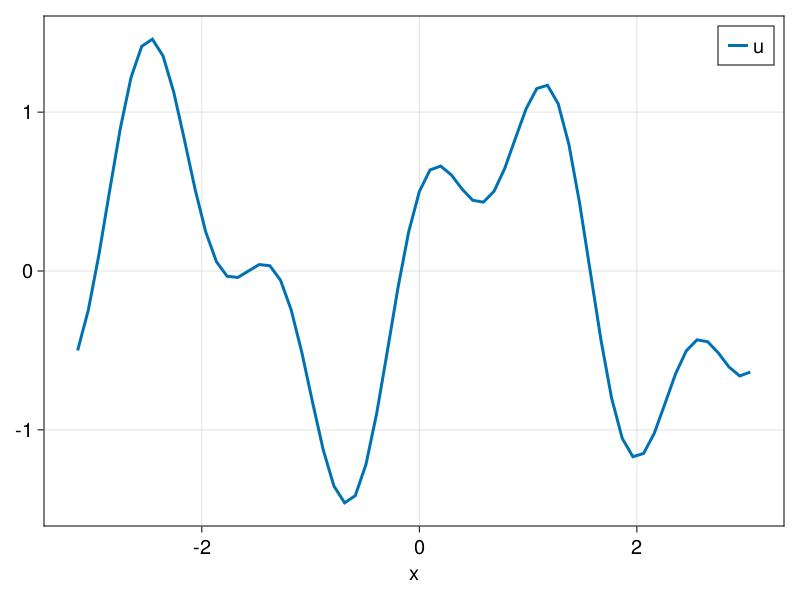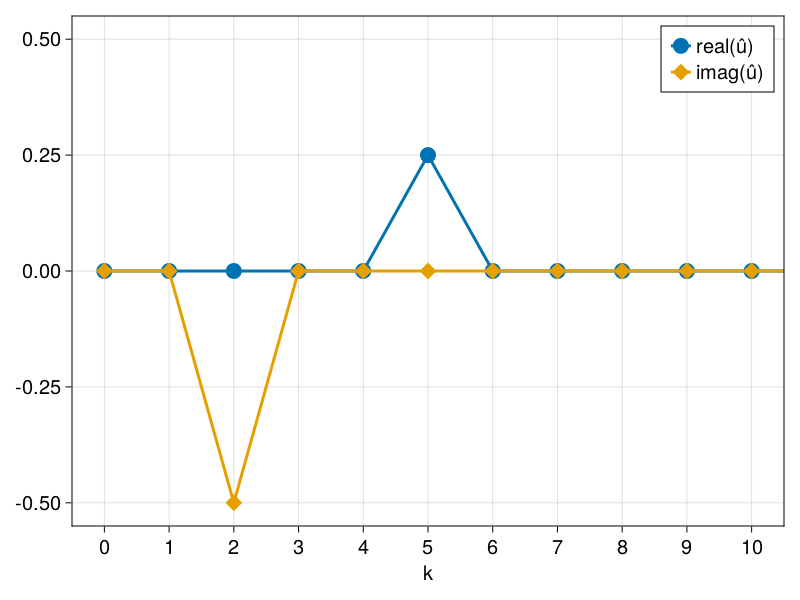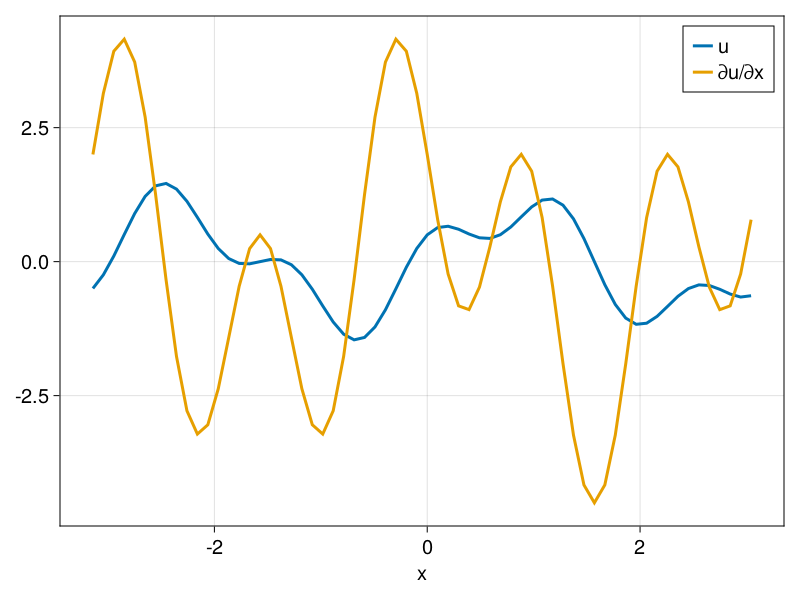# Grids

1D, 2D, and 3D grids are supported. We demonstrate here the construction of a one-dimensional grid and how one can use it to perform Fourier transforms and compute spatial derivatives.

A one-dimensional grid with $n_x = 64$ grid points and length $L_x = 2 \pi$ is constructed by

using FourierFlows

nx, Lx = 64, 2π

grid = OneDGrid(nx, Lx)

# output

OneDimensionalGrid
├─────────── Device: CPU
├──────── FloatType: Float64
├────────── size Lx: 6.283185307179586
├──── resolution nx: 64
├── grid spacing dx: 0.09817477042468103
├─────────── domain: x ∈ [-3.141592653589793, 3.0434178831651124]
└─ aliased fraction: 0.3333333333333333

The grid domain is, by default, constructed symmetrically around $x = 0$, but this can be altered using the x0 keyword argument of the OneDGrid constructor. The grid spacing is $L_x / n_x$. Note that the last point of the domain is a grid-spacing before $L_x / 2$. This is because periodicity implies that the values of any field at the end-points of the domain are equal and, therefore, grid-point values at both these end-points are redundant.

We can define an array u that contains the values of a function $u(x)$ on this grid as

u = @. sin(2 * grid.x) + 1/2 * cos(5 * grid.x)

Note that we chose a function that is periodic on our domain. We can visualize u by

using CairoMakie

lines(grid.x, u, label="u", axis = (xlabel="x",))
axislegend()Function $u(x)$ can be expanded in Fourier series:

$$$u(x) = \sum_{k} \hat{u}(k) \, e^{i k x} ,$$$

where $\hat{u}(k)$ is Fourier transform of $u(x)$ and $k$ the discrete set of wavenumbers that fit within our finite domain. We can compute $\hat{u}$ via a Fast Fourier Transform (FFT). Since our u array is real-valued then we should use the real-FFT algorithm. The real-valued FFT transform only saves the Fourier coefficients for $k \ge 0$; the coefficients for negative wavenumbers can be obtained via $\hat{u}(-k) = \hat{u}(k)^{*}$.

The wavenumbers used in FFT are contained in grid.k and they are ordered as:

$$$\frac{2\pi}{L_x} \{ 0, 1, \dots, n_x/2-1, -n_x/2, -n_x/2+1, \dots, -1 \} ,$$$

while the wavenumbers for real FFT are in grid.kr:

$$$\frac{2\pi}{L_x} \{ 0, 1, \dots, n_x/2-1 \} .$$$

The grid also includes the FFT plans for both real-valued and complex valued transforms:

grid.fftplan
FFTW forward plan for 64-element array of ComplexF64
(dft-direct-64 "n2fv_64_avx2_128")
grid.rfftplan
FFTW real-to-complex plan for 64-element array of Float64
(rdft2-ct-dit/2
(hc2c-direct-2/4/1 "hc2cfdftv_2_avx2"
(rdft2-r2hc-direct-2 "r2cf_2")
(rdft2-r2hc01-direct-2 "r2cfII_2"))
(dft-direct-32 "n2fv_32_avx2_128"))

We use the convention that variables names with h at the end stand for variable-hat, i.e., $\hat{u}$ is the Fourier transform of $u$ and is stored in array uh. Since u is of size $n_x$, the real-Fourier transform should be of size $n_{kr} = n_x/2+1$.

uh = Complex.(zeros(grid.nkr))

The FFT transform is done as an in-place matrix multiplication using mul!.

using LinearAlgebra: mul!

mul!(uh, grid.rfftplan, u)

The FFT algorithm does not output exactly the Fourier coefficients $\hat{u}(k)$ but rather, due to different normalization, FFT outputs something proportional to $\hat{u}(k)$. To obtain $\hat{u}$ we need to divide the FFT output by the length of the original array and by $e^{-i k x_0}$, where $x_0$ is the first point of our domain array.

uhat = @. uh / (nx * exp(- im * grid.kr * grid.x)) # due to normalization of FFT

fig = Figure()
ax = Axis(fig[1, 1];
xlabel = "k",
limits = ((-0.5, 10.5), (-0.55, 0.55)),
xticks = 0:10,
yticks = -0.5:0.25:0.5)

scatterlines!(ax, grid.kr, real.(uhat);
markersize = 16, label = "real(û)")

scatterlines!(ax, grid.kr, imag.(uhat);
markersize = 22, marker = :diamond, label = "imag(û)")

axislegend()We can compute the derivative of $u(x)$ via Fourier transforms. To do that we use the FFTW plans that are constructed with the grid. First we allocate some empty arrays where the values of the derivative will be stored, both in physical and in Fourier space:

∂ₓu  = similar(u)
∂ₓuh = similar(uh)

The grid contains the wavenumbers (both for real-value functions grid.kr and for complex-valued functions grid.k). We populate array ∂ₓuh is with $i k \hat{u}$:

@. ∂ₓuh = im * grid.kr * uh

Then the derivative in physical space, ∂ₓu, is obtained with an inverse Fourier transform. The latter is obtained again using the FFTW plans but now via ldiv!:

using LinearAlgebra: ldiv!

ldiv!(∂ₓu, grid.rfftplan, ∂ₓuh)

fig = Figure()
ax = Axis(fig[1, 1], xlabel = "x")

lines!(ax, grid.x, u, label = "u")
lines!(ax, grid.x, ∂ₓu, label = "∂u/∂x")

axislegend()